## Saturday, July 30, 2011

### DCF Can Lead To Large Mistakes!

Saw the following posting on Nakedcapitalism.

Some points to highlight...

• In calculating this average, some paths turn out to contribute far more than others. In particular, paths that descend into relatively low rates and stay there for many years have a disproportionate effect — a path at 1 percent for 50 years, for instance, counts 20 times as much as a path running along at 7 percent. Change 50 to 500 years, and the difference becomes 10 trillion times.

This demonstrates how simple thinking about the future can lead to terrific mistakes

Let me reproduce the postings again...
1.  DCF (Discounted Cash Flow) example

I remembered this Wallstraits write-up on
GHL Systems way back in May 2003.

Here is a snippet of what they wrote back then.

• Valuation Simulation This is not a forecast or recommendation
GHL is likely to experience close to 100% growth again in the current year, 2003, and then growth will slow, we will assume to a sustainable 20% annual rate over the next nine years in this simulation. Current year (2003) full-year net cash flow from operations is estimated at RM 0.04 per share (adding RM 3.5m depreciation back to RM 6.5m net earnings-- RM 10m CF / 250m shares = RM 0.04). We will also assume a 5% discount rate (US Treasury risk free rate) and no terminal value of the business after year 10.
•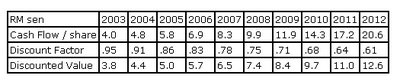• Given these assumptions (you may want to recalculate this simulation using your own cash flow, growth and discount rate assumptions), GHL’s intrinsic business value based on discounted cash flow expected to be produced from 2003 to 2012 is RM 74.5, which is approximately a 72% discount to the current market share price of around RM 0.21.

PE check: If GHL does achieve cash flow per share of 8.3 sen in 2007 (5-years forward), and at that time GHL is valued by the market at 10-times cash flow, the share price would need to appreciate from 21 sen today to 83 sen, or an appreciation of nearly 300% in 5-years. Of course, GHL has only been listed on Mesdaq for a month, so our assumptions will likely need refinement as the quarters and years pass.
Firstly, do remember that this was a stimulation only done by Wallstraits.com.

Anyway, I reckon that what interests you is how they did the cash flow table. Below is their table again:Ok let me share with you my understanding of what is being done by Wallstraits.

1. Cash Flow.
First of all, for 2003, that 4.0 sen cash flow is derived from the following manner according to Wallstraits.
• Current year (2003) full-year net cash flow from operations is estimated at RM 0.04 per share (adding RM 3.5m depreciation back to RM 6.5m net earnings-- RM 10m CF / 250m shares = RM 0.04).
So they added the 3.5 million depreciation back to their projected earnings of 6.5 million. Which will equal to 10 million. Divide that by 250 million shares, you would get a cash flow per share of 4 sen for 2003.

(What could go wrong here? The projected earnings of 6.5 million. Just for the record GHL did about 6.0 million (see here ))

2. DF or Discount Factor is assumed at 5%.

So a 5% discount of 1 would equal 0.95

(So far, a 5% discount factor still seems to be fair... but if the interest rates
were to increase some more... it would disrupt the whole table)

3. DV or Discounted value

The discounted Value = 4.0 x 0.95 = 3.80

Next column. We need to remember the next assumption made by Wallstraits.
• we will assume to a sustainable 20% annual rate over the next nine years in this simulation.
1. Cash Flow.
In 2003, the calculated cash flow is at 4 sen. A 20% annual growth rate would see the cash flow increase by 4.0 x 20% = 4.8 sen.

2. DF
The discount factor was set at 5%. So the discount factor for 2004 = 0.95 less 5% = 0.91 (rounded up)

3. DV
The DV equals 4.8 * 0.91 = 4.36 (or 4.4 rounded up).
and so on... and so on...

So what could go wrong in these series of calculations?

the starting point of course. The staring point or the initial projected cash flow is utmost important. Put in an optimistic starting value, and you would get a rather optmistic end result.

the annual growth rate. Here Wallstraits assumes a 20% annual growth rate. Try a different growth rate, and you would get a total different value.

the discount rate. here it is assumed to be 5%. what if there is a drastic change in the midst of this time frame?

hope this helps...

cheers!

---------------------
2. Using the DCF

Dedicated to farnaway:

Using the same stimulation done by Wallstraits on GHL.

First of all here is Wallstraits table again.And here is their interpretation of the table:

• Given these assumptions (you may want to recalculate this simulation using your own cash flow, growth and discount rate assumptions), GHL’s intrinsic business value based on discounted cash flow expected to be produced from 2003 to 2012 is RM 74.5, which is approximately a 72% discount to the current market share price of around RM 0.21.
The total discounted value is calculated by adding all the sum of the DV from 2003 to 2012. Which they get a value of 74.5 sen per share. And when one compare this value to the market price of GHL in May 2003 of 21 sen, one would see that it is trading at a discount of 72%.

And from a Price earnings perspective, this is what Wallstraits had to say...

• If GHL does achieve cash flow per share of 8.3 sen in 2007 (5-years forward), and at that time GHL is valued by the market at 10-times cash flow, the share price would need to appreciate from 21 sen today to 83 sen, or an appreciation of nearly 300% in 5-years. Of course, GHL has only been listed on Mesdaq for a month, so our assumptions will likely need refinement as the quarters and years pass.
Sounds fair, right?

now GHL latest quarterly earnings announced in Feb saw it reporting a net profit of 11.656 million. Depreciation was reported to be 6.368 million. Giving GHL a free cash flow of 18.024 million. And if one continued to use Wallstrait numbers, ie number of GHL shares at 250 million, this would equate to a free cash flow of 7.2 sen. And what was Wallstraits numbers? 5.8 sen. How?

And to complicate matters, GHL had a series of 1 for 4 bonus issue and also a 3 for 5 rights issue. All in which bloated the current number of shares to 551.480 million shares. And based on the enlarged share base, GHL free cash flow should be 3.3 sen for its fiscal year 2005.

Now, if i redo the whole table... using 10 million as the starting total cash flow and based on 551.480 million shares, the starting cash flow should be 1.8 sen.

And here is how the table should look like.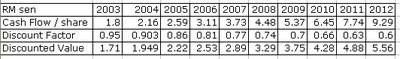Let's look at the 2005 results. The stimulation showed cash flow per share to be at 2.59 sen. Actual? 3.3 sen.

Here's more intresting stuff... create a same table under Excel and play with some numbers...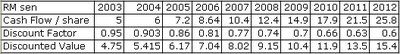The above was set using the initial cash flow at 5 sen. End result? total discounted cash flow f rom 2003 to 2012 would work out to 91.85 sen. (compare to 74.5). And at 2007, total cash flow is at 10.4 sen. (compare to wallstraits 8.3 sen).

Now let me change the starting point to 3 sen. And the below is the end result.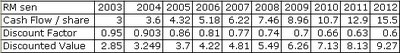Total cash flow from 2003 to 2012 equals 55.11 (compare t0 74.5 sen) and 2007 cash flow per share is at 6.22 sen (compare to 8,3 sen). See how much difference it makes by changing the starting terminal point?

Ok. How about changing the annual growth rate? remember Wallstraits used 20% per annum.

Here is how the table would look like using a 25% growth rate and using 4 sen as the intial starting point.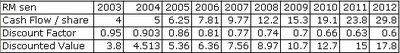The total discounted cash flow from 2003 to 2012 would now total 92.74 (compare to 74.5) and 2007 cash flow would equal 9.77 sen (compare to 8.3 sen)

Or how about just a 12% per annum growth rate instead of 20%?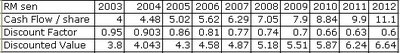End result? Total discounted cash flow would now total 51.03 sen (compare to 74.5) and 2007 cash flow per share is only 6.29 sen (compare to 8.3 sen)

See how complicated things get?

Ahhh... I could continue by changing the discounted factor... and the end result would differ greatly.

Hope this posting helps!

Cheers!

------------------------------------------

And how is GHL System doing?

As you can see, GHL is losing money since 2008.

************************************

Here's another good recommended posting: http://cgmalaysia.blogspot.com/2011/07/abolish-dcf-models-in-circulars.html

K C said...

DCF is the foundation in valuation in finance. However it depends on some artistic assumptions, discount rate and growth rates are the major ones. Wrongly used will be GIGO (garbage in garbage out). I think the valuation of GHL in your posting is GIGO. Firstly how can the risk free rate be used as a discount rate? Are you investing in Treasury bills or Cagamas bond or what? I would at least at 5% risk premium to the risk free rate, or 10% minimum as a discount rate, or what my required return is. Secondly, I presume the valuation should be based on free cash flow (FCF). FCF is not obtained from adding back non-cash item as depreciation alone to earnings. You need additional working capital when the business grows. The more important is the capital expenditures. The valuation in GHL has no capital expenditures? How can a company grow so fast without any capex? A company can initially grow at 20% for 2,3 or even 5 years. But continuously growing at 20% for 10 years way too liberal. This growth rate has really boost up the FCF many folds in 10 years. In my opinion, all assumptions used are garbage.

Moolah said...

I agree and that is why my title is DCF CAN lead to large mistakes.

And yes, the whole assumptions is garbage.

But having said that, that's the whole point, isn't it?

Take the research reports that uses DCF.

How many care to show the reader how they come up with their DCF calculation? What's the assumptions use?

M.A. Wind said...

Very good post, completely agree, I wrote about it in my blog:

http://cgmalaysia.blogspot.com/2011/07/abolish-dcf-models-in-circulars.html

K C said...

M.A. Wind,
Despite the pitfalls in DCF, I do not agree that finance should do away with it. It is the "correct" way to value a business, even though it is theoretical. How do you know what is the worth of a business? Earnings and book value can be and are usually managed like the infamous Enron and thousands more companies. What is the probable range of intrinsic value of the business? What is the expected IRR? What is the margin of safety if one were to invest in it(W Buffet)? It is just that the assumptions must be realistic. The GHL case mentioned contain assumptions that are all highly unrealistic and utterly wrong for the sole purpose of trying to jack up the share price. That was a deliberate misuse of DCF.

Moolah said...

K C: I would say I say agree with M. Wind. It's not against DCF itself but it's how research reports give their fair value based on DCF. Like I said, how many of these reports care to show to the readers how they come up with their DCF calculations? What's the assumptions use?

You must understand why I chose GHL. It was one of the articles posted online back then which actually showed how they used DCF to calculate their fair value for GHL. And all I did was mess around here and there, just to show how 'complicated' things could be. A small change of discount rate could see a drastic change in value and we all know if we discounted it on a longer time frame, the values gets really... err.... distorted.

Singapore Man of Leisure said...

Hello Moolah,

Assumption is to guess. And no need to "guess" 10 years forward. Try guessing the next quarter!

By using DCF in analyst's reports, they give an "illusion" of precision and certainty to investors. Surely the numbers don't lie!?

People somehow like to believe in numbers - but they forget to ask who "manipulated" the numbers in the first place!